SSC CGL Previous Year Questions: Simplification and Square & Cube Root- 4

# SSC CGL Previous Year Questions: Simplification and Square & Cube Root- 4 - SSC CGL

Test Description

## 20 Questions MCQ Test SSC CGL (Tier - 1) - Previous Year Papers (Topic Wise) - SSC CGL Previous Year Questions: Simplification and Square & Cube Root- 4

SSC CGL Previous Year Questions: Simplification and Square & Cube Root- 4 for SSC CGL 2023 is part of SSC CGL (Tier - 1) - Previous Year Papers (Topic Wise) preparation. The SSC CGL Previous Year Questions: Simplification and Square & Cube Root- 4 questions and answers have been prepared according to the SSC CGL exam syllabus.The SSC CGL Previous Year Questions: Simplification and Square & Cube Root- 4 MCQs are made for SSC CGL 2023 Exam. Find important definitions, questions, notes, meanings, examples, exercises, MCQs and online tests for SSC CGL Previous Year Questions: Simplification and Square & Cube Root- 4 below.
Solutions of SSC CGL Previous Year Questions: Simplification and Square & Cube Root- 4 questions in English are available as part of our SSC CGL (Tier - 1) - Previous Year Papers (Topic Wise) for SSC CGL & SSC CGL Previous Year Questions: Simplification and Square & Cube Root- 4 solutions in Hindi for SSC CGL (Tier - 1) - Previous Year Papers (Topic Wise) course. Download more important topics, notes, lectures and mock test series for SSC CGL Exam by signing up for free. Attempt SSC CGL Previous Year Questions: Simplification and Square & Cube Root- 4 | 20 questions in 20 minutes | Mock test for SSC CGL preparation | Free important questions MCQ to study SSC CGL (Tier - 1) - Previous Year Papers (Topic Wise) for SSC CGL Exam | Download free PDF with solutions
 1 Crore+ students have signed up on EduRev. Have you?
SSC CGL Previous Year Questions: Simplification and Square & Cube Root- 4 - Question 1

###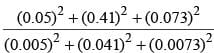is       (SSC CGL 2011)

Detailed Solution for SSC CGL Previous Year Questions: Simplification and Square & Cube Root- 4 - Question 1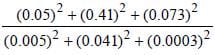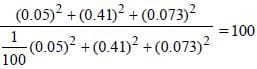SSC CGL Previous Year Questions: Simplification and Square & Cube Root- 4 - Question 2

### The value of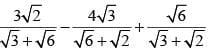is       (SSC CGL 2011)

Detailed Solution for SSC CGL Previous Year Questions: Simplification and Square & Cube Root- 4 - Question 2

Expression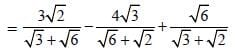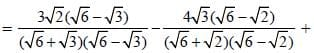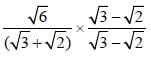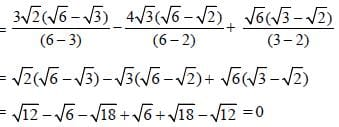SSC CGL Previous Year Questions: Simplification and Square & Cube Root- 4 - Question 3

### The value of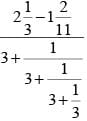is       (SSC CGL 1st Sit. 2011)

Detailed Solution for SSC CGL Previous Year Questions: Simplification and Square & Cube Root- 4 - Question 3

Expression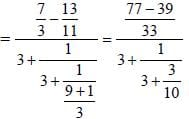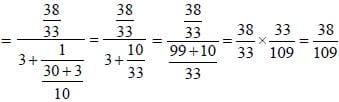SSC CGL Previous Year Questions: Simplification and Square & Cube Root- 4 - Question 4

The square root of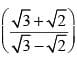is       (SSC CGL  1st Sit. 2011)

Detailed Solution for SSC CGL Previous Year Questions: Simplification and Square & Cube Root- 4 - Question 4

Expression =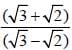Rationalising the denominator,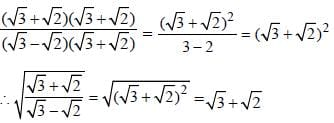SSC CGL Previous Year Questions: Simplification and Square & Cube Root- 4 - Question 5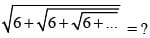(SSC CGL 1st Sit. 2011)

Detailed Solution for SSC CGL Previous Year Questions: Simplification and Square & Cube Root- 4 - Question 5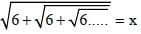6 = 3 × 2
By trick = 3

SSC CGL Previous Year Questions: Simplification and Square & Cube Root- 4 - Question 6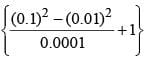is equal to       (SSC CGL 2nd Sit. 2010)

Detailed Solution for SSC CGL Previous Year Questions: Simplification and Square & Cube Root- 4 - Question 6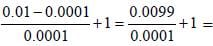99 + 1 = 100

SSC CGL Previous Year Questions: Simplification and Square & Cube Root- 4 - Question 7

Out of six consecutive natural numbers, if the sum of first three is 27, what is the sum of the other three ?     (SSC CGL 2nd Sit. 2010)

Detailed Solution for SSC CGL Previous Year Questions: Simplification and Square & Cube Root- 4 - Question 7

8 + 9 + 10 = 27
11 + 12 + 13 = 36
So, let 3 consecutive no x, x + 1, x + 2
Next 3 consecutive no x + 3; x + 4, x + 5
i.e. sum of last 3 consecutive no. is 9 more than sum of first 3.
= 27 + 9 = 36

SSC CGL Previous Year Questions: Simplification and Square & Cube Root- 4 - Question 8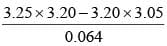is equal to      (SSC CGL 2nd Sit. 2010)

Detailed Solution for SSC CGL Previous Year Questions: Simplification and Square & Cube Root- 4 - Question 8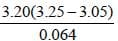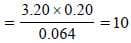SSC CGL Previous Year Questions: Simplification and Square & Cube Root- 4 - Question 9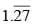in the form p/q is equal to      (SSC CGL 2st Sit. 2010)

Detailed Solution for SSC CGL Previous Year Questions: Simplification and Square & Cube Root- 4 - Question 9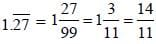SSC CGL Previous Year Questions: Simplification and Square & Cube Root- 4 - Question 10

The least among the fractions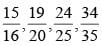is       (SSC CGL 2nd Sit. 2010)

Detailed Solution for SSC CGL Previous Year Questions: Simplification and Square & Cube Root- 4 - Question 10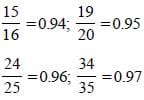SSC CGL Previous Year Questions: Simplification and Square & Cube Root- 4 - Question 11

(0.1 × 0.01 × 0.001 × 107) is equal to        (SSC CGL 2nd Sit. 2010)

Detailed Solution for SSC CGL Previous Year Questions: Simplification and Square & Cube Root- 4 - Question 11

0.1 × 0.01 × 0.001 × 107 = 10–6 × 107 = 10

SSC CGL Previous Year Questions: Simplification and Square & Cube Root- 4 - Question 12

Simplified form of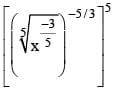is       (SSC CGL 2nd Sit. 2010)

Detailed Solution for SSC CGL Previous Year Questions: Simplification and Square & Cube Root- 4 - Question 12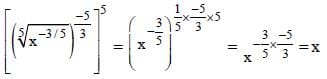SSC CGL Previous Year Questions: Simplification and Square & Cube Root- 4 - Question 13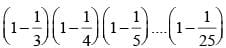is equal to       (SSC CGL 2nd Sit. 2010)

Detailed Solution for SSC CGL Previous Year Questions: Simplification and Square & Cube Root- 4 - Question 13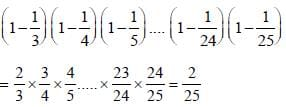SSC CGL Previous Year Questions: Simplification and Square & Cube Root- 4 - Question 14

(12 + 22 + 32 + ...... + 102) is equal to       (SSC CGL 2nd Sit. 2010)

Detailed Solution for SSC CGL Previous Year Questions: Simplification and Square & Cube Root- 4 - Question 14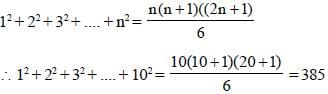SSC CGL Previous Year Questions: Simplification and Square & Cube Root- 4 - Question 15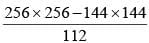is equal to        (SSC CGL 2nd Sit. 2010)

Detailed Solution for SSC CGL Previous Year Questions: Simplification and Square & Cube Root- 4 - Question 15

If 256 = a and 144 = b, then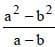[a – b = 256 – 144 = 112]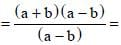a + b = 256 + 144 = 400

SSC CGL Previous Year Questions: Simplification and Square & Cube Root- 4 - Question 16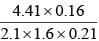is simplified to        (SSC CGL 2nd Sit. 2010)

Detailed Solution for SSC CGL Previous Year Questions: Simplification and Square & Cube Root- 4 - Question 16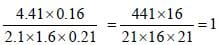SSC CGL Previous Year Questions: Simplification and Square & Cube Root- 4 - Question 17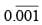is equal to       (SSC CGL 2nd Sit. 2010)

Detailed Solution for SSC CGL Previous Year Questions: Simplification and Square & Cube Root- 4 - Question 17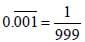SSC CGL Previous Year Questions: Simplification and Square & Cube Root- 4 - Question 18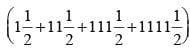is equal to       (SSC CGL 2nd Sit. 2010)

Detailed Solution for SSC CGL Previous Year Questions: Simplification and Square & Cube Root- 4 - Question 18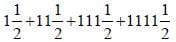= 1234 + 2 = 1236

SSC CGL Previous Year Questions: Simplification and Square & Cube Root- 4 - Question 19

By what least number should  675 be multiplied so as to obtain a perfect cube number ?        (SSC CGL 2nd Sit. 2010)

Detailed Solution for SSC CGL Previous Year Questions: Simplification and Square & Cube Root- 4 - Question 19

675 = 5 × 5 × 3 × 3 × 3 = 5
No. to be multiplied

SSC CGL Previous Year Questions: Simplification and Square & Cube Root- 4 - Question 20

The number 0.121212.... in the form p/q is equal to       (SSC CGL 1st Sit. 2010)

Detailed Solution for SSC CGL Previous Year Questions: Simplification and Square & Cube Root- 4 - Question 20

0.121212... =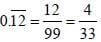## SSC CGL (Tier - 1) - Previous Year Papers (Topic Wise)

250 tests
Information about SSC CGL Previous Year Questions: Simplification and Square & Cube Root- 4 Page
In this test you can find the Exam questions for SSC CGL Previous Year Questions: Simplification and Square & Cube Root- 4 solved & explained in the simplest way possible. Besides giving Questions and answers for SSC CGL Previous Year Questions: Simplification and Square & Cube Root- 4, EduRev gives you an ample number of Online tests for practice

250 tests